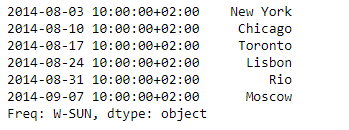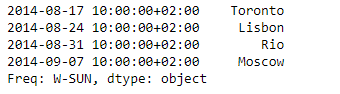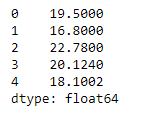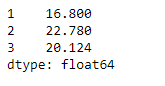# Python | Pandas Series.truncate()

• Last Updated : 05 Feb, 2019

Pandas series is a One-dimensional ndarray with axis labels. The labels need not be unique but must be a hashable type. The object supports both integer- and label-based indexing and provides a host of methods for performing operations involving the index.

Pandas` Series.truncate()` function is used to truncate a Series or DataFrame before and after some index value. This is a useful shorthand for boolean indexing based on index values above or below certain thresholds.

Syntax: Series.truncate(before=None, after=None, axis=None, copy=True)

Parameter :
before : Truncate all rows before this index value.
after : Truncate all rows after this index value.
axis : Axis to truncate. Truncates the index (rows) by default.
copy : Return a copy of the truncated section.

Returns : truncated Series or DataFrame.

Example #1: Use `Series.truncate()` function to truncate some data from the series prior to a given date.

 `# importing pandas as pd ` `import` `pandas as pd ` ` `  `# Creating the Series ` `sr ``=` `pd.Series([``'New York'``, ``'Chicago'``, ``'Toronto'``, ``'Lisbon'``, ``'Rio'``, ``'Moscow'``]) ` ` `  `# Create the Datetime Index ` `didx ``=` `pd.DatetimeIndex(start ``=``'2014-08-01 10:00'``, freq ``=``'W'``,  ` `                     ``periods ``=` `6``, tz ``=` `'Europe/Berlin'``)  ` ` `  `# set the index ` `sr.index ``=` `didx ` ` `  `# Print the series ` `print``(sr) `

Output :Now we will use `Series.truncate()` function to truncate data which are prior to ‘2014-08-17 10:00:00+02:00’ in the given Series object.

 `# truncate data prior to the given date ` `sr.truncate(before ``=` `'2014-08-17 10:00:00 + 02:00'``) `

Output :As we can see in the output, the `Series.truncate()` function has successfully truncated all data prior to the mentioned date.

Example #2: Use `Series.truncate()` function to truncate some data from the series prior to a given index label and after a given index label.

 `# importing pandas as pd ` `import` `pandas as pd ` ` `  `# Creating the Series ` `sr ``=` `pd.Series([``19.5``, ``16.8``, ``22.78``, ``20.124``, ``18.1002``]) ` ` `  `# Print the series ` `print``(sr) `

Output :Now we will use `Series.truncate()` function to truncate data which are prior to the 1st index label and after the 3rd index label in the given Series object.

 `# truncate data outside the given range ` `sr.truncate(before ``=` `1``, after ``=` `3``) `

Output :As we can see in the output, the `Series.truncate()` function has successfully truncated all data prior to the mentioned index label and after the mentioned index label.

My Personal Notes arrow_drop_up
Recommended Articles
Page :